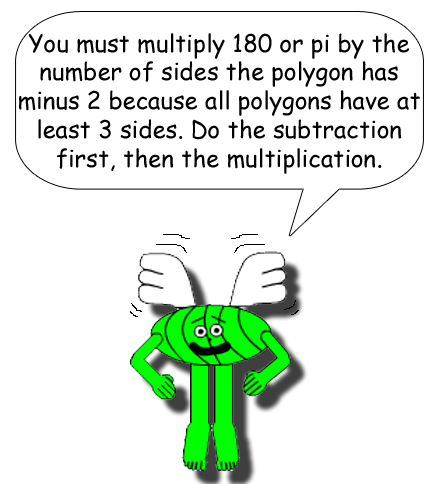The table below shows what the sum of the internal angles of a polygon always add up to. The prefix before -gon tells you how many sides the polygon has(if the number is greater than 4). Most prefixes (& suffixes) are from the Greek & Latin languages. One column of the table will give you a clue to which number the prefix stands for.

Degrees & radians both measure angles, but radians are based on the formula for the circumference of a circle. Degrees are based on rotation. When it comes to polygons, people usually consider degrees when calculating the sizes of the internal angles, but if you convert the sum of the angles from degrees to radians, something quite interesting happens! Look below to see!Triangle3180π
Pentagon5540
Hexagon6720
Heptagon7900
Octagon81,080
Nonagon91,260
Decagon101,440
Undecagon111,620
Dodecagon121,80010π
X-gonX(X - 2)180(X - 2)π

Have you noticed something about the radians? π is multiplied by consecutive integers as you scroll down the rows! Why? Because 360 degrees = 2π radians. That also means 180 degrees = π radians, so to convert degrees to radians, multiply by π/180; multiply by 180/π to convert radians to degrees.

Note: To find out the average angle size of a polygon, simply divide the sum of degrees/radians by the number of angles/sides.

You should already know that multiplying(or dividing) a number by 1 gives you the same number. That's because 1 is the number of multiplicative identity.To conclude this subject, remember that there's always 1 opposite angle for each side of a polygon. In other words, the number of angles & the number of sides are always equal!